Examples

Chapter 11 Class 12 Three Dimensional Geometry
Serial order wise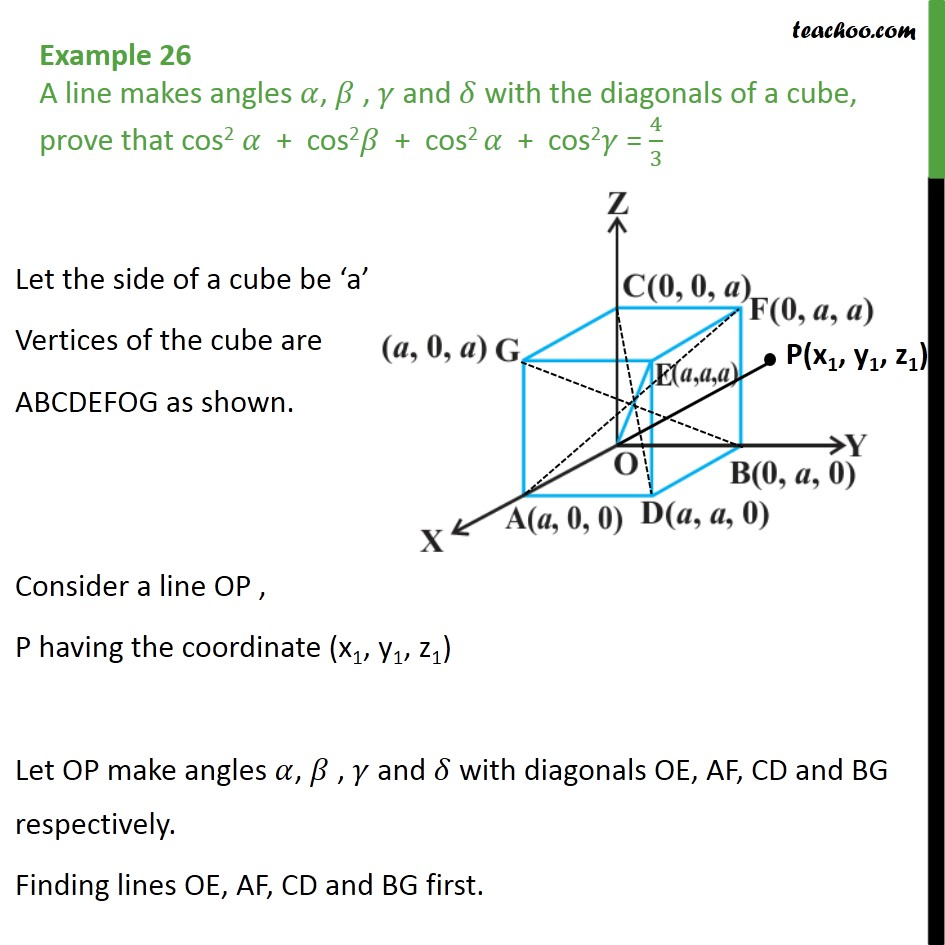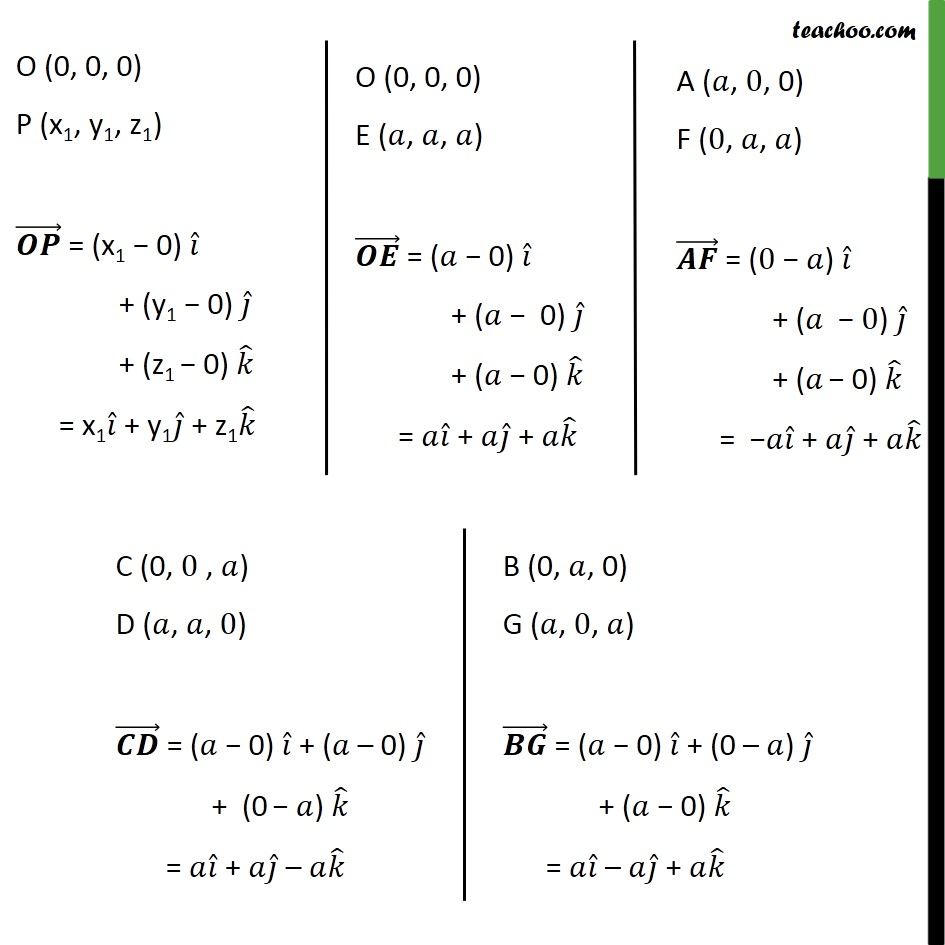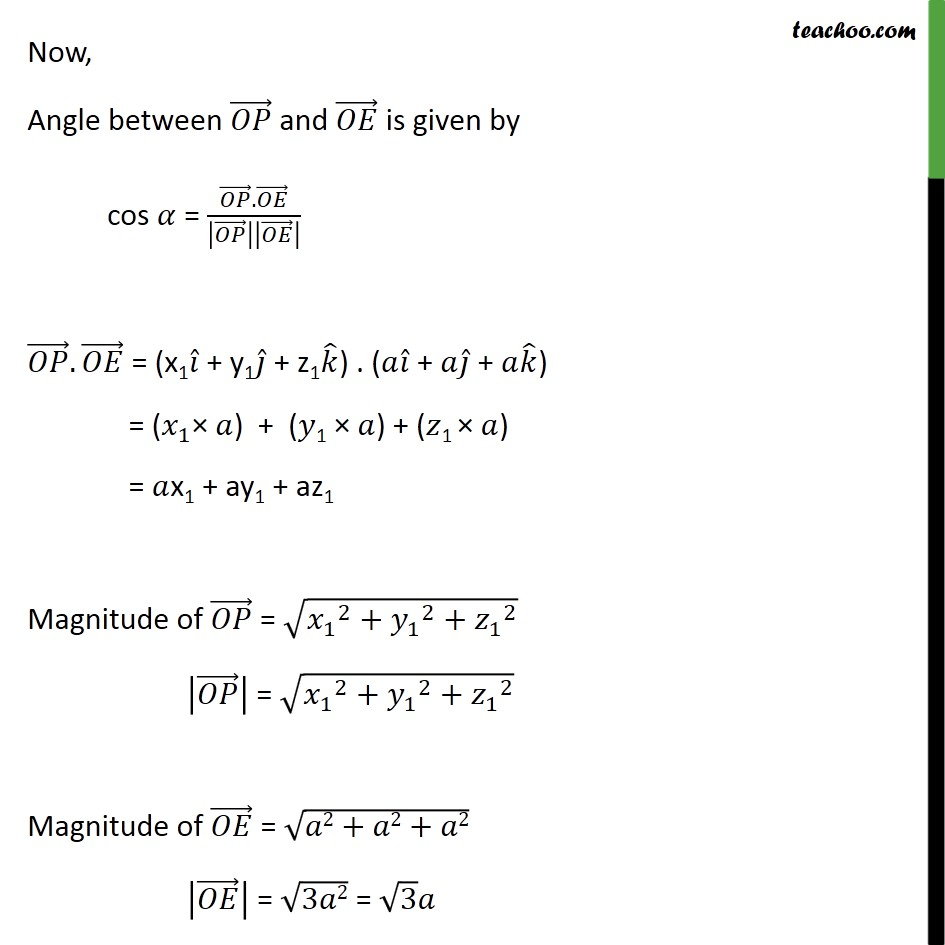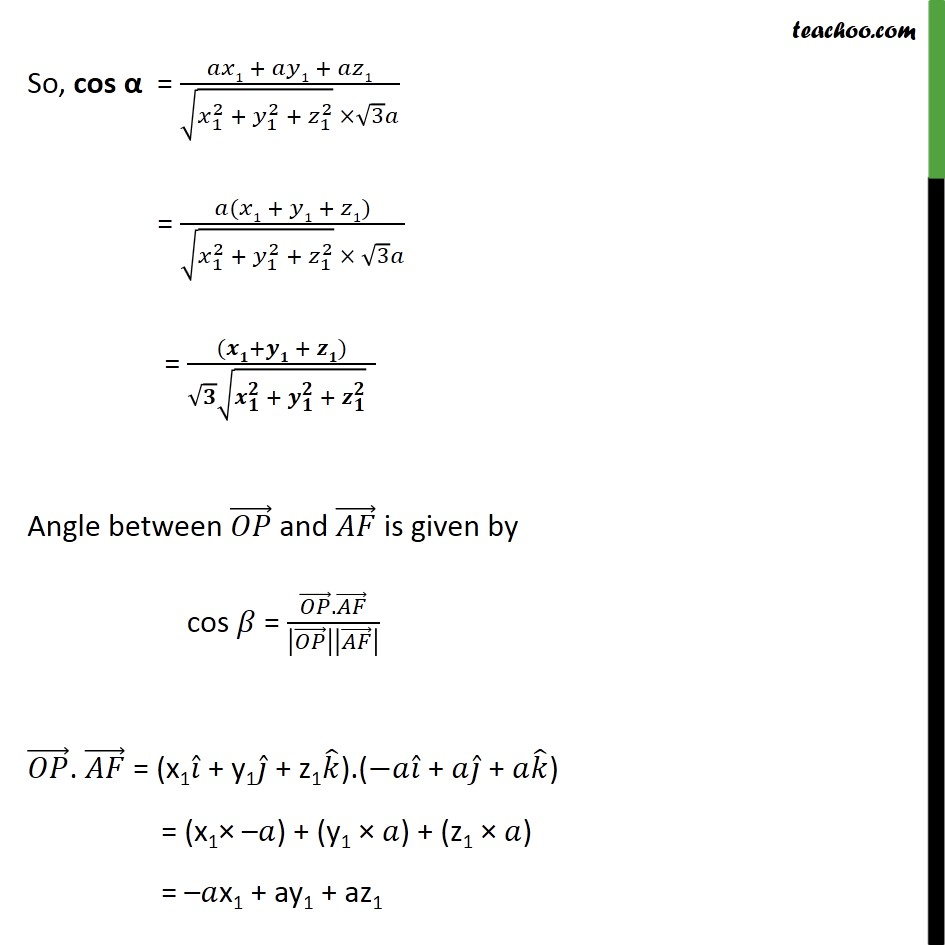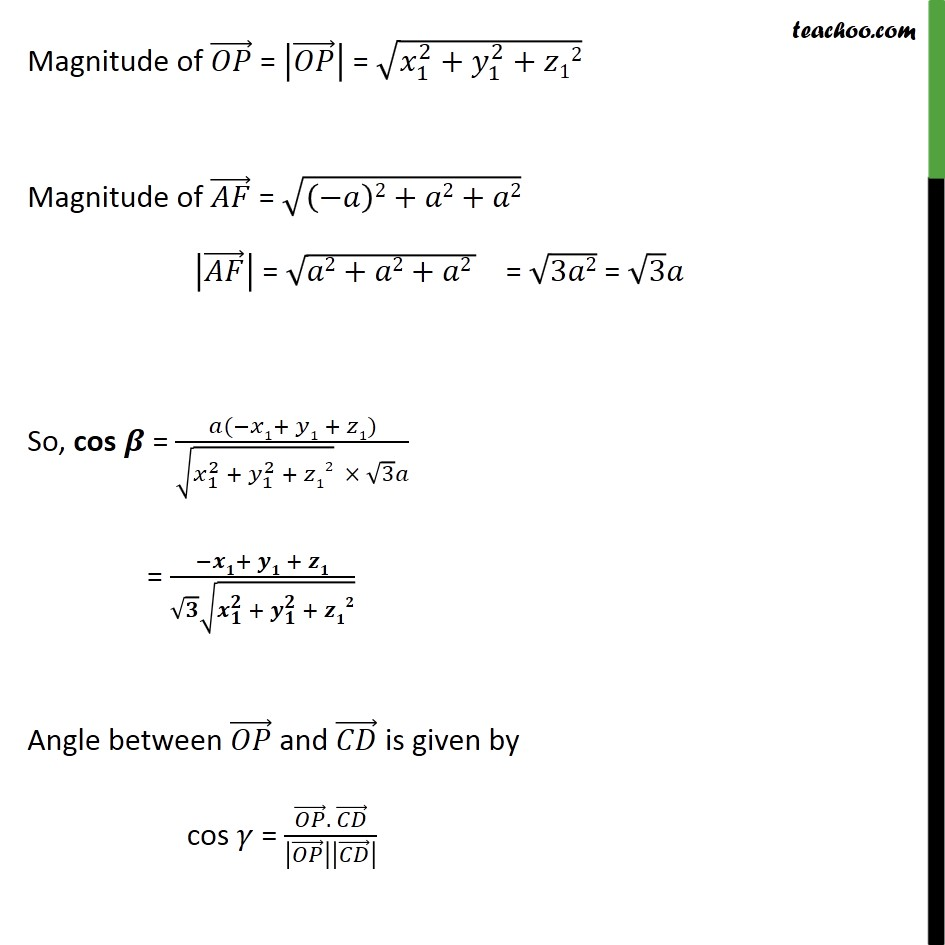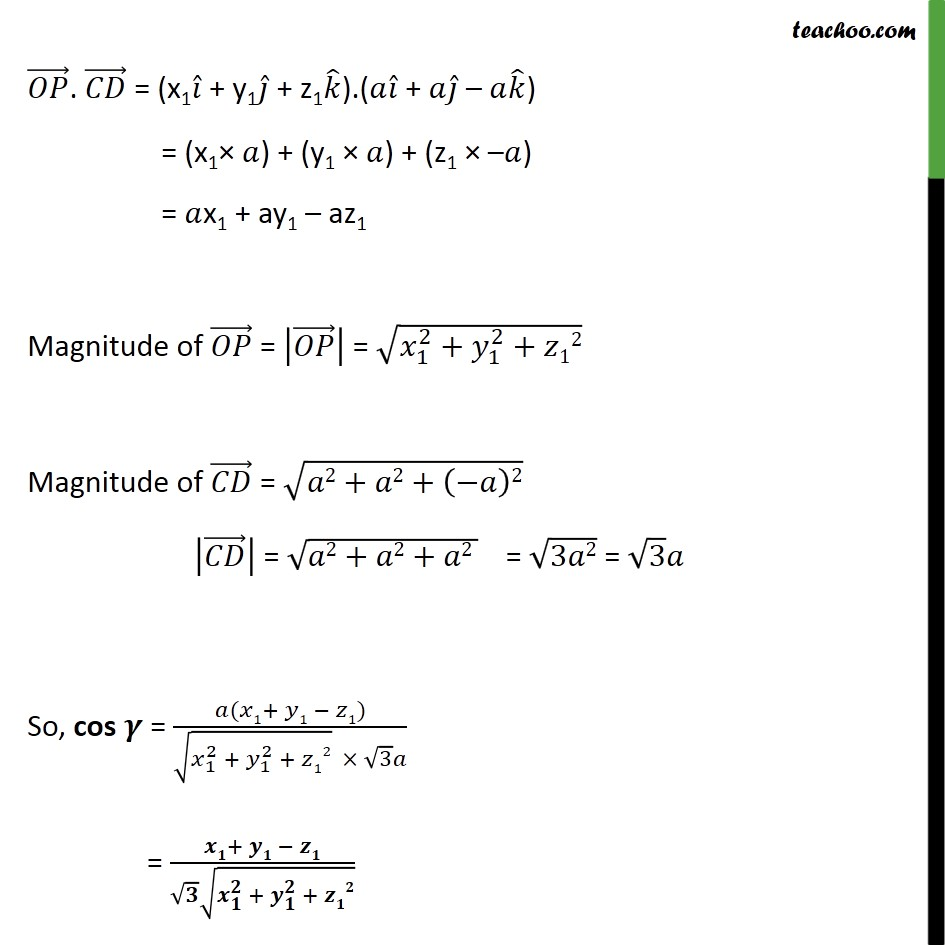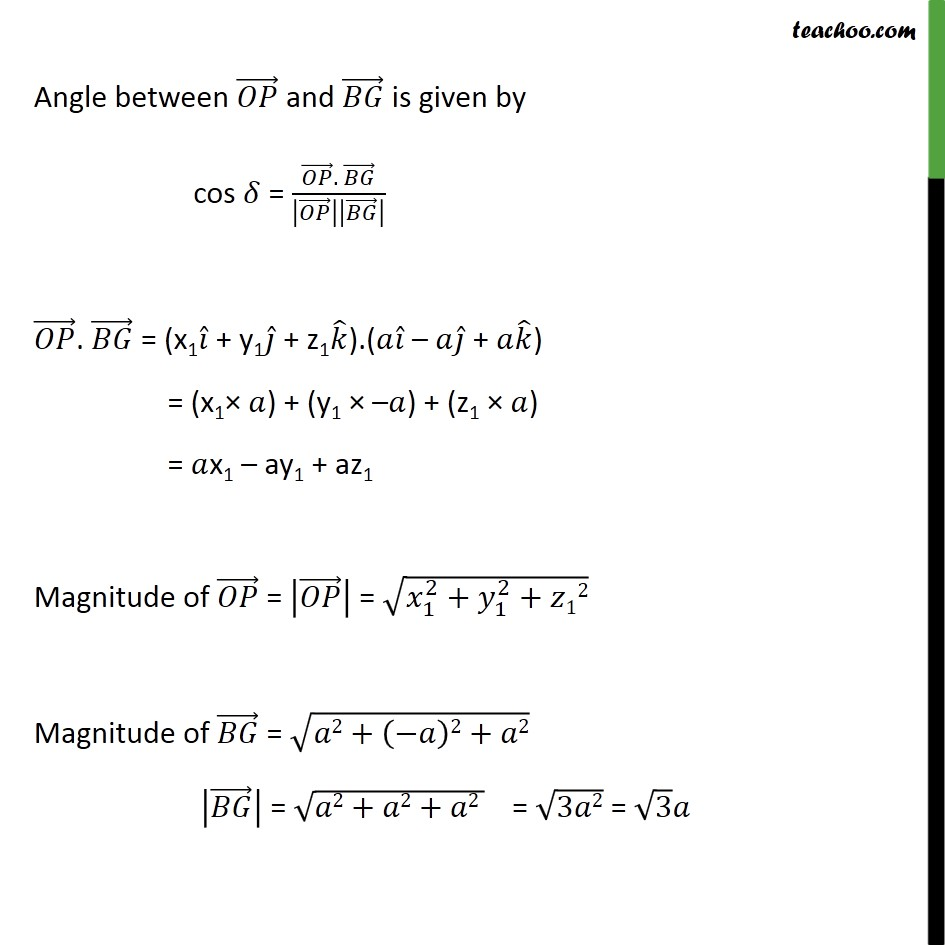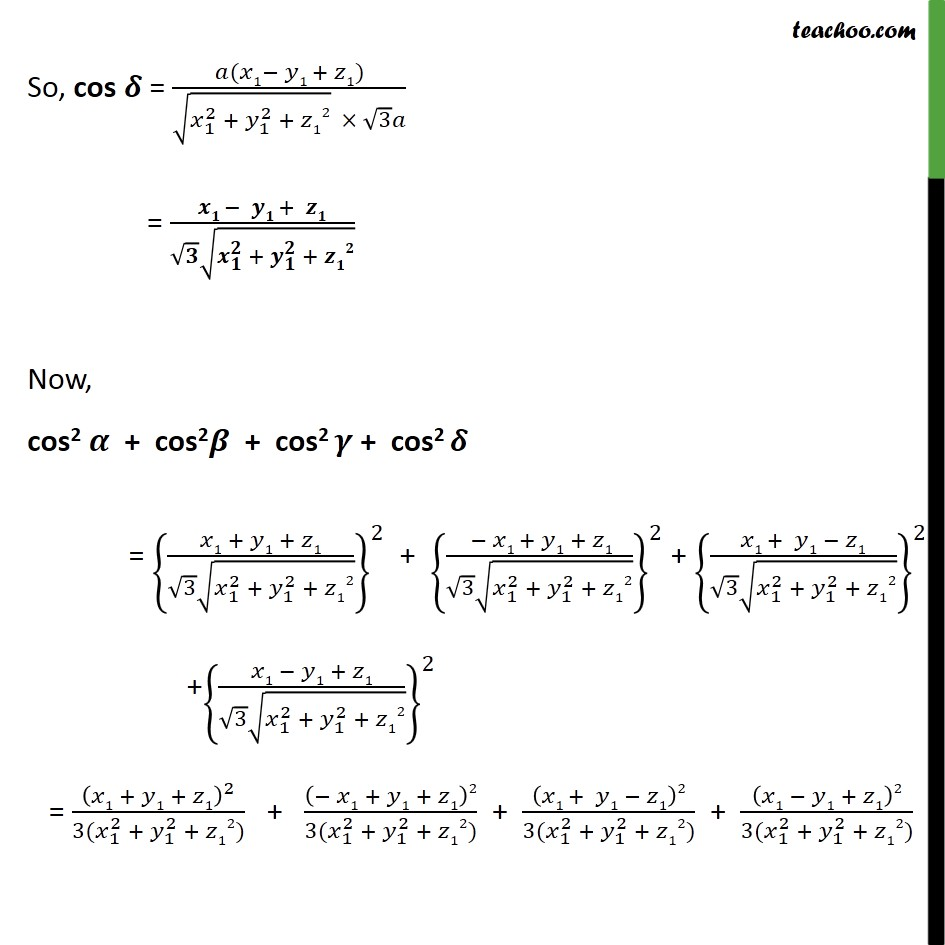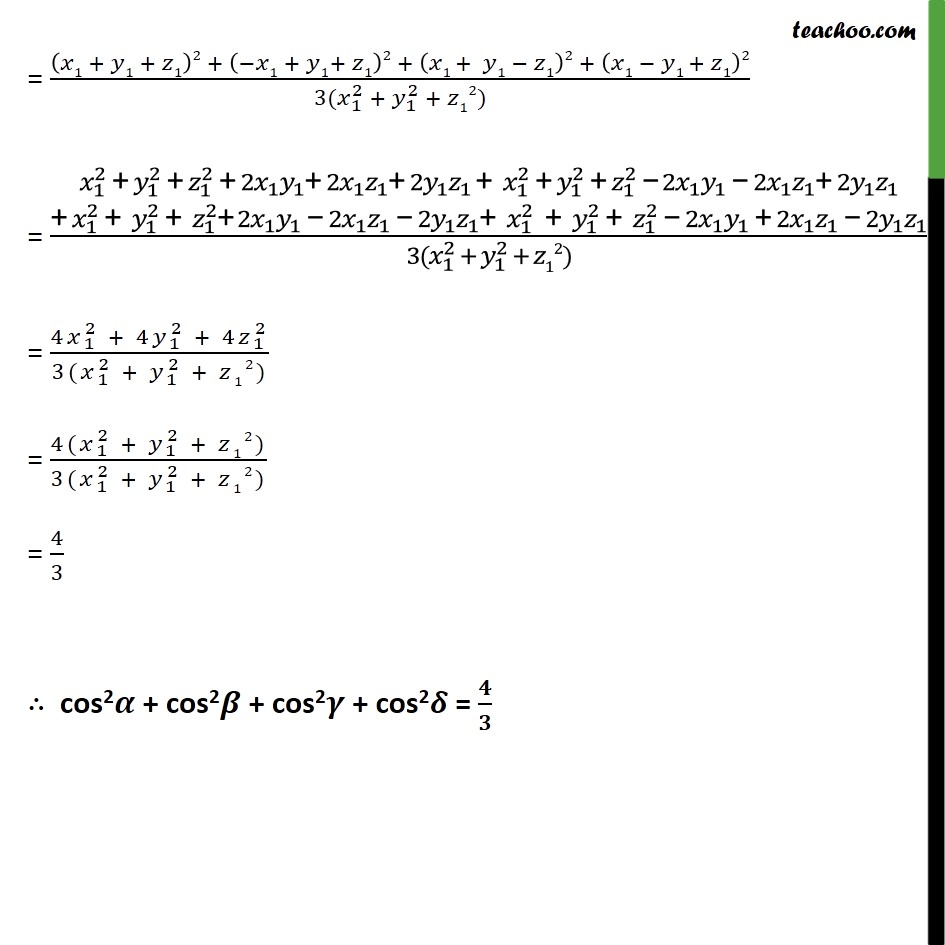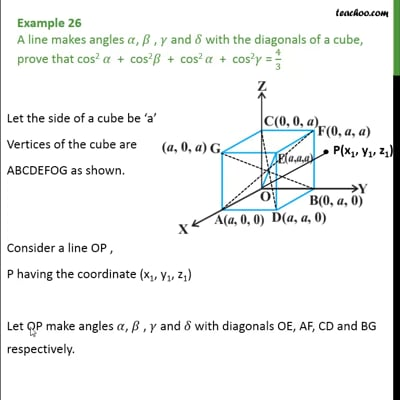This video is only available for Teachoo black users

Maths Crash Course - Live lectures + all videos + Real time Doubt solving!

### Transcript

Example 26 A line makes angles 𝛼, 𝛽 , 𝛾 and 𝛿 with the diagonals of a cube, prove that cos2 𝛼 + cos2𝛽 + cos2 𝛼 + cos2𝛾 = 4﷮3﷯ Let the side of a cube be ‘a’ Vertices of the cube are ABCDEFOG as shown. Consider a line OP , P having the coordinate (x1, y1, z1) Let OP make angles 𝛼, 𝛽 , 𝛾 and 𝛿 with diagonals OE, AF, CD and BG respectively. Finding lines OE, AF, CD and BG first. Now, Angle between 𝑂𝑃﷯ and 𝑂𝐸﷯ is given by cos 𝛼 = 𝑂𝑃﷯. 𝑂𝐸﷯﷮ 𝑂𝑃﷯﷯ 𝑂𝐸﷯﷯﷯ 𝑂𝑃﷯. 𝑂𝐸﷯ = (x1 𝑖﷯ + y1 𝑗﷯ + z1 𝑘﷯) . (𝑎 𝑖﷯ + 𝑎 𝑗﷯ + 𝑎 𝑘﷯) = ( 𝑥﷮1﷯× 𝑎) + (𝑦1 × 𝑎) + (𝑧1 × 𝑎) = 𝑎x1 + ay1 + az1 Magnitude of 𝑂𝑃﷯ = ﷮ 𝑥﷮1﷯﷮2﷯+ 𝑦﷮1﷯﷮2﷯+ 𝑧﷮1﷯﷮2﷯﷯ 𝑂𝑃﷯﷯ = ﷮ 𝑥﷮1﷯﷮2﷯+ 𝑦﷮1﷯﷮2﷯+ 𝑧﷮1﷯﷮2﷯﷯ Magnitude of 𝑂𝐸﷯ = ﷮𝑎2+𝑎2+𝑎2﷯ 𝑂𝐸﷯﷯ = ﷮3𝑎2﷯ = ﷮3﷯𝑎 So, cos α = 𝑎𝑥1 + 𝑎𝑦1 + 𝑎𝑧1﷮ ﷮ 𝑥﷮1﷮2﷯ + 𝑦﷮1﷮2﷯ + 𝑧﷮1﷮2﷯﷯ × ﷮3﷯𝑎﷯ = 𝑎(𝑥1 + 𝑦1 + 𝑧1)﷮ ﷮ 𝑥﷮1﷮2﷯ + 𝑦﷮1﷮2﷯ + 𝑧﷮1﷮2﷯﷯ × ﷮3﷯𝑎﷯ = (𝒙𝟏+𝒚𝟏 + 𝒛𝟏)﷮ ﷮𝟑﷯ ﷮ 𝒙﷮𝟏﷮𝟐﷯ + 𝒚﷮𝟏﷮𝟐﷯ + 𝒛﷮𝟏﷮𝟐﷯﷯ ﷯ Angle between 𝑂𝑃﷯ and 𝐴𝐹﷯ is given by cos 𝛽 = 𝑂𝑃﷯. 𝐴𝐹﷯﷮ 𝑂𝑃﷯﷯ 𝐴𝐹﷯﷯﷯ 𝑂𝑃﷯. 𝐴𝐹﷯ = (x1 𝑖﷯ + y1 𝑗﷯ + z1 𝑘﷯).(−𝑎 𝑖﷯ + 𝑎 𝑗﷯ + 𝑎 𝑘﷯) = (x1× –𝑎) + (y1 × 𝑎) + (z1 × 𝑎) = –𝑎x1 + ay1 + az1 Magnitude of 𝑂𝑃﷯ = 𝑂𝑃﷯﷯ = ﷮ 𝑥﷮1﷮2﷯+ 𝑦﷮1﷮2﷯+𝑧12﷯ Magnitude of 𝐴𝐹﷯ = ﷮ −𝑎﷯2+𝑎2+𝑎2﷯ 𝐴𝐹﷯﷯ = ﷮𝑎2+𝑎2+𝑎2 ﷯ = ﷮3𝑎2﷯ = ﷮3﷯𝑎 So, cos 𝜷 = 𝑎(−𝑥1+ 𝑦1 + 𝑧1)﷮ ﷮ 𝑥﷮1﷮2﷯ + 𝑦﷮1﷮2﷯ + 𝑧12﷯ × ﷮3﷯𝑎﷯ = −𝒙𝟏+ 𝒚𝟏 + 𝒛𝟏﷮ ﷮𝟑﷯ ﷮ 𝒙﷮𝟏﷮𝟐﷯ + 𝒚﷮𝟏﷮𝟐﷯ + 𝒛𝟏𝟐﷯﷯ Angle between 𝑂𝑃﷯ and 𝐶𝐷﷯ is given by cos 𝛾 = 𝑂𝑃﷯. 𝐶𝐷﷯﷮ 𝑂𝑃﷯﷯ 𝐶𝐷﷯﷯﷯ 𝑂𝑃﷯. 𝐶𝐷﷯ = (x1 𝑖﷯ + y1 𝑗﷯ + z1 𝑘﷯).(𝑎 𝑖﷯ + 𝑎 𝑗﷯ – 𝑎 𝑘﷯) = (x1× 𝑎) + (y1 × 𝑎) + (z1 × –𝑎) = 𝑎x1 + ay1 – az1 Magnitude of 𝑂𝑃﷯ = 𝑂𝑃﷯﷯ = ﷮ 𝑥﷮1﷮2﷯+ 𝑦﷮1﷮2﷯+𝑧12﷯ Magnitude of 𝐶𝐷﷯ = ﷮𝑎2+𝑎2+ −𝑎﷯2﷯ 𝐶𝐷﷯﷯ = ﷮𝑎2+𝑎2+𝑎2 ﷯ = ﷮3𝑎2﷯ = ﷮3﷯𝑎 So, cos 𝜸 = 𝑎(𝑥1+ 𝑦1 − 𝑧1)﷮ ﷮ 𝑥﷮1﷮2﷯ + 𝑦﷮1﷮2﷯ + 𝑧12﷯ × ﷮3﷯𝑎﷯ = 𝒙𝟏+ 𝒚𝟏 − 𝒛𝟏﷮ ﷮𝟑﷯ ﷮ 𝒙﷮𝟏﷮𝟐﷯ + 𝒚﷮𝟏﷮𝟐﷯ + 𝒛𝟏𝟐﷯﷯ Angle between 𝑂𝑃﷯ and 𝐵𝐺﷯ is given by cos 𝛿 = 𝑂𝑃﷯. 𝐵𝐺﷯﷮ 𝑂𝑃﷯﷯ 𝐵𝐺﷯﷯﷯ 𝑂𝑃﷯. 𝐵𝐺﷯ = (x1 𝑖﷯ + y1 𝑗﷯ + z1 𝑘﷯).(𝑎 𝑖﷯ – 𝑎 𝑗﷯ + 𝑎 𝑘﷯) = (x1× 𝑎) + (y1 × –𝑎) + (z1 × 𝑎) = 𝑎x1 – ay1 + az1 Magnitude of 𝑂𝑃﷯ = 𝑂𝑃﷯﷯ = ﷮ 𝑥﷮1﷮2﷯+ 𝑦﷮1﷮2﷯+𝑧12﷯ Magnitude of 𝐵𝐺﷯ = ﷮𝑎2+ −𝑎﷯2+𝑎2﷯ 𝐵𝐺﷯﷯ = ﷮𝑎2+𝑎2+𝑎2 ﷯ = ﷮3𝑎2﷯ = ﷮3﷯𝑎 So, cos 𝜹 = 𝑎(𝑥1− 𝑦1 + 𝑧1)﷮ ﷮ 𝑥﷮1﷮2﷯ + 𝑦﷮1﷮2﷯ + 𝑧12﷯ × ﷮3﷯𝑎﷯ = 𝒙𝟏 − 𝒚𝟏 + 𝒛𝟏﷮ ﷮𝟑﷯ ﷮ 𝒙﷮𝟏﷮𝟐﷯ + 𝒚﷮𝟏﷮𝟐﷯ + 𝒛𝟏𝟐﷯﷯ Now, cos2 𝜶 + cos2𝜷 + cos2 𝜸 + cos2 𝜹 = 𝑥1 + 𝑦1 + 𝑧1﷮ ﷮3﷯ ﷮ 𝑥﷮1﷮2﷯ + 𝑦﷮1﷮2﷯ + 𝑧12﷯﷯﷯﷮2﷯ + − 𝑥1 + 𝑦1 + 𝑧1﷮ ﷮3﷯ ﷮ 𝑥﷮1﷮2﷯ + 𝑦﷮1﷮2﷯ + 𝑧12﷯﷯﷯﷮2﷯ + 𝑥1 + 𝑦1 − 𝑧1﷮ ﷮3﷯ ﷮ 𝑥﷮1﷮2﷯ + 𝑦﷮1﷮2﷯ + 𝑧12﷯﷯﷯﷮2﷯ + 𝑥1 − 𝑦1 + 𝑧1﷮ ﷮3﷯ ﷮ 𝑥﷮1﷮2﷯ + 𝑦﷮1﷮2﷯ + 𝑧12﷯﷯﷯﷮2﷯ = 𝑥1 + 𝑦1 + 𝑧1﷯﷮2﷯﷮3( 𝑥﷮1﷮2﷯ + 𝑦﷮1﷮2﷯ + 𝑧12)﷯ + − 𝑥1 + 𝑦1 + 𝑧1﷯2﷮3( 𝑥﷮1﷮2﷯ + 𝑦﷮1﷮2﷯ + 𝑧12)﷯ + 𝑥1 + 𝑦1 − 𝑧1﷯2﷮3( 𝑥﷮1﷮2﷯ + 𝑦﷮1﷮2﷯ + 𝑧12)﷯ + 𝑥1 − 𝑦1 + 𝑧1﷯2﷮3( 𝑥﷮1﷮2﷯ + 𝑦﷮1﷮2﷯ + 𝑧12)﷯ = 𝑥1 + 𝑦1 + 𝑧1﷯2 + −𝑥1 + 𝑦1+ 𝑧1﷯2 + 𝑥1 + 𝑦1 − 𝑧1﷯2 + 𝑥1 − 𝑦1 + 𝑧1﷯2﷮3( 𝑥﷮1﷮2﷯ + 𝑦﷮1﷮2﷯ + 𝑧12)﷯ = 𝑥﷮1﷮2﷯ + 𝑦﷮1﷮2﷯ + 𝑧﷮1﷮2﷯ + 2 𝑥﷮1﷯ 𝑦﷮1﷯+ 2 𝑥﷮1﷯ 𝑧﷮1﷯+ 2 𝑦﷮1﷯ 𝑧﷮1﷯ + 𝑥﷮1﷮2﷯ + 𝑦﷮1﷮2﷯ + 𝑧﷮1﷮2﷯ − 2 𝑥﷮1﷯ 𝑦﷮1﷯ − 2 𝑥﷮1﷯ 𝑧﷮1﷯+ 2 𝑦﷮1﷯ 𝑧﷮1﷯﷮+ 𝑥﷮1﷮2﷯ + 𝑦﷮1﷮2﷯ + 𝑧﷮1﷮2﷯+ 2 𝑥﷮1﷯ 𝑦﷮1﷯ − 2 𝑥﷮1﷯ 𝑧﷮1﷯ − 2 𝑦﷮1﷯ 𝑧﷮1﷯+ 𝑥﷮1﷮2﷯ + 𝑦﷮1﷮2﷯ + 𝑧﷮1﷮2﷯ − 2 𝑥﷮1﷯ 𝑦﷮1﷯ + 2 𝑥﷮1﷯ 𝑧﷮1﷯ − 2 𝑦﷮1﷯ 𝑧﷮1﷯﷯﷮3( 𝑥﷮1﷮2﷯ + 𝑦﷮1﷮2﷯ + 𝑧12)﷯ = 4 𝑥﷮1﷮2﷯ + 4 𝑦﷮1﷮2﷯ + 4 𝑧﷮1﷮2﷯﷮3( 𝑥﷮1﷮2﷯ + 𝑦﷮1﷮2﷯ + 𝑧12)﷯ = 4( 𝑥﷮1﷮2﷯ + 𝑦﷮1﷮2﷯ + 𝑧12)﷮3( 𝑥﷮1﷮2﷯ + 𝑦﷮1﷮2﷯ + 𝑧12)﷯ = 4﷮3﷯ ∴ cos2𝜶 + cos2𝜷 + cos2𝜸 + cos2𝜹 = 𝟒﷮𝟑﷯